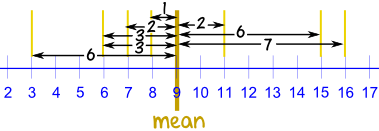Standard

# Mean

The mean is the average of the numbers.

It is easy to calculate: add up all the numbers, then divide by how many numbers there are.

# Absolute Value

Absolute Value means …

… only how far a number is from zero:“6” is 6 away from zero, and “−6” is also 6 away from zero. So the absolute value of 6 is 6, and the absolute value of −6 is also 6

# Mean Absolute Deviation

Mean absolute deviation (MAD) is about calculating the average distance from the mean.

Click here to watch a video from our 8th Grade Math textbook that goes in to detail and explain how to calculate the MAD.

MEAN ABSOLUTE DEVIATION:

The average/mean distance from the average/mean is the MAD.

Yes, we use “mean” twice: Find the mean … use it to work out distances … then find the mean of those!

### Three steps:

• 1. Find the mean of all values
• 2. Find the distance of each value from that mean (subtract the mean from each value, ignore negative signs)
• 3. Then find the mean of those distances

Like this:

### 3, 6, 6, 7, 8, 11, 15, 16

Step 1: Find the mean:

 Mean = 3 + 6 + 6 + 7 + 8 + 11 + 15 + 16 = 72 = 9 8 8

Step 2: Find the distance of each value from that mean:

Value Distance from 9
3 6
6 3
6 3
7 2
8 1
11 2
15 6
16 7

Which looks like this:Step 3. Find the mean of those distances:

 Mean Deviation = 6 + 3 + 3 + 2 + 1 + 2 + 6 + 7 = 30 = 3.75 8 8

So, the mean = 9, and the mean deviation = 3.75

It tells us how far, on average, all values are from the middle.

In that example the values are, on average, 3.75 away from the middle.

### For deviation just think distance

MEAN ABSOLUTE DEVIATION Practice Problems:

## Random Sample

This is where everyone has an equal and fair chance of being selected.